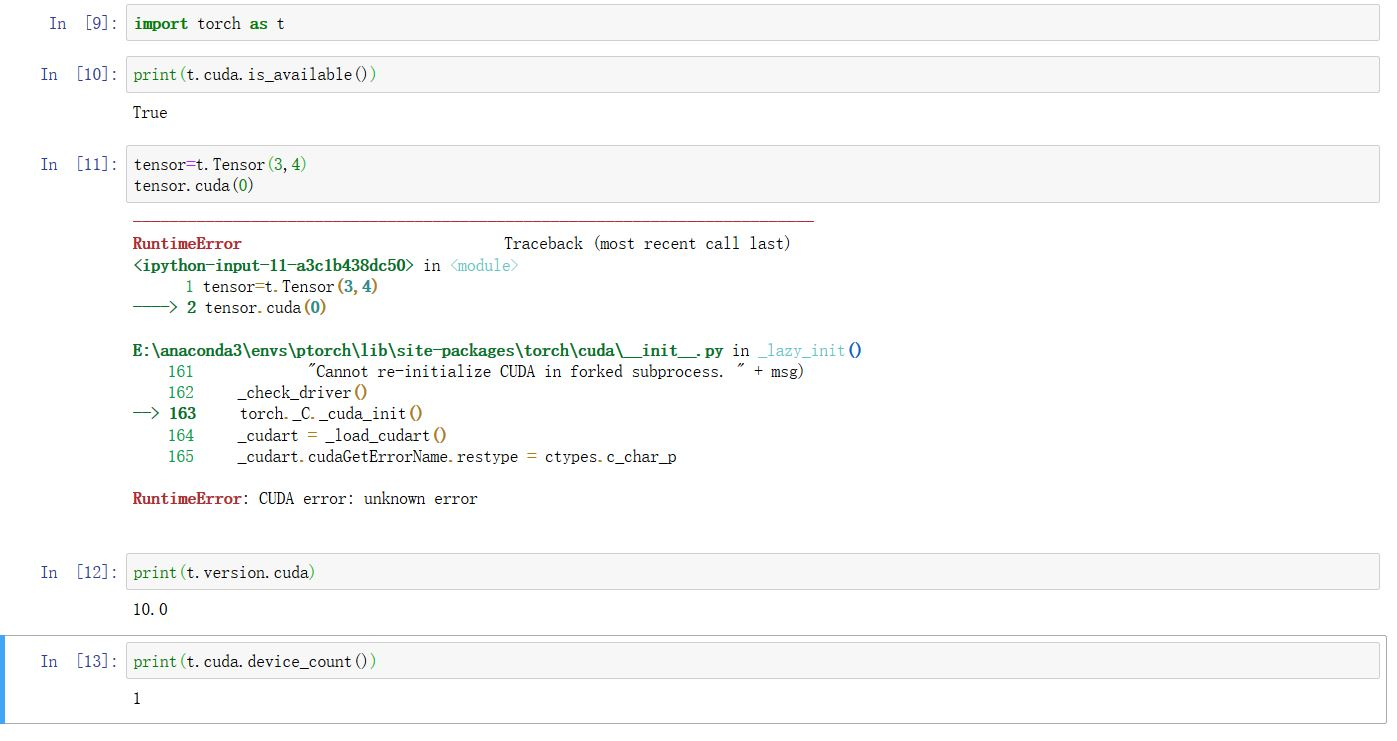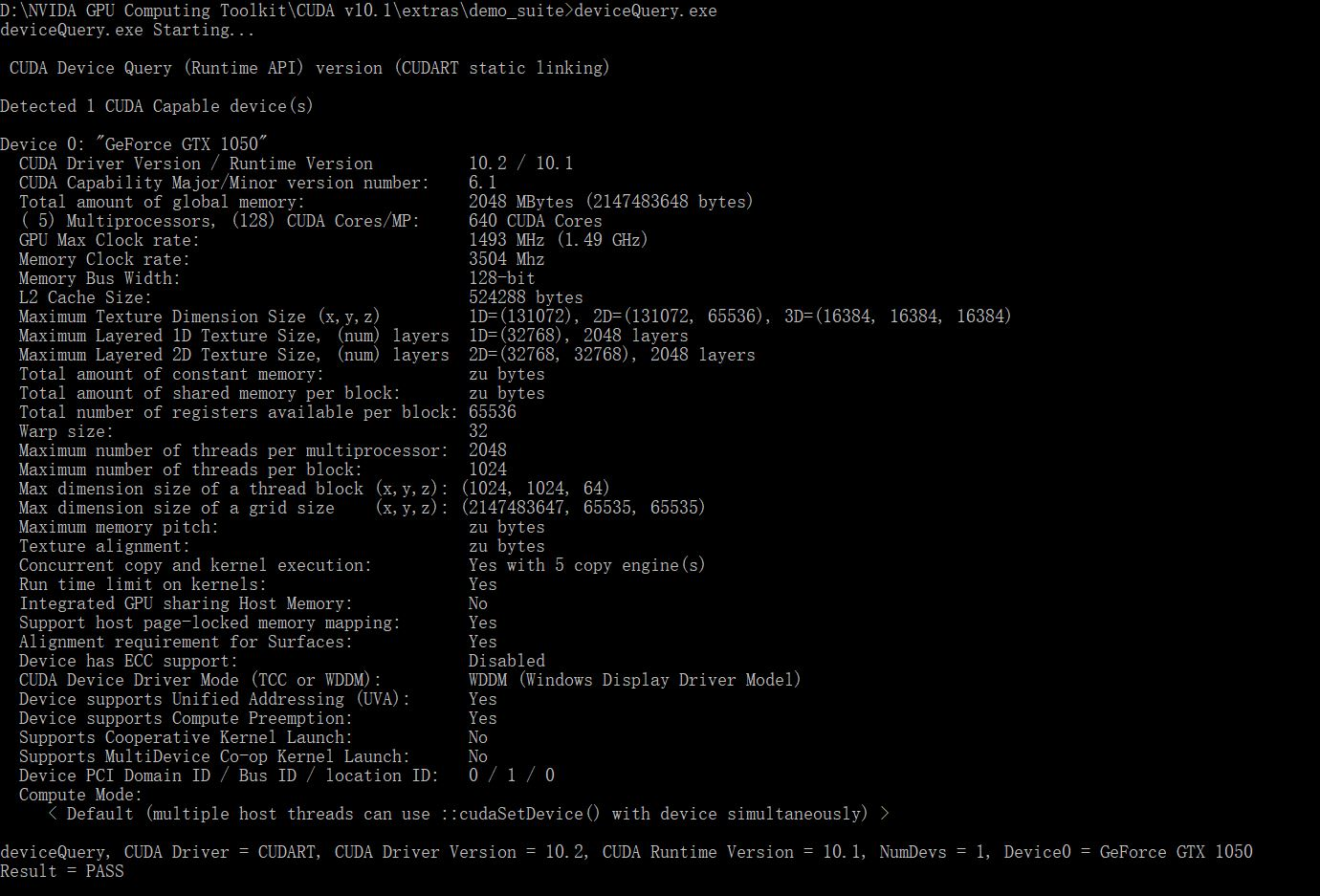pytorch使用cuda报错RuntimeError: CUDA error: unknown error，如何解决？cuda可用但是把tensor放到gpu上就会报这个错

``````import torch as t
tensor=t.Tensor(3,4)
tensor.cuda(0)
````````````import torch as t
tensor=t.Tensor(3,4)
tensor.cuda(0)
``````

3个回答